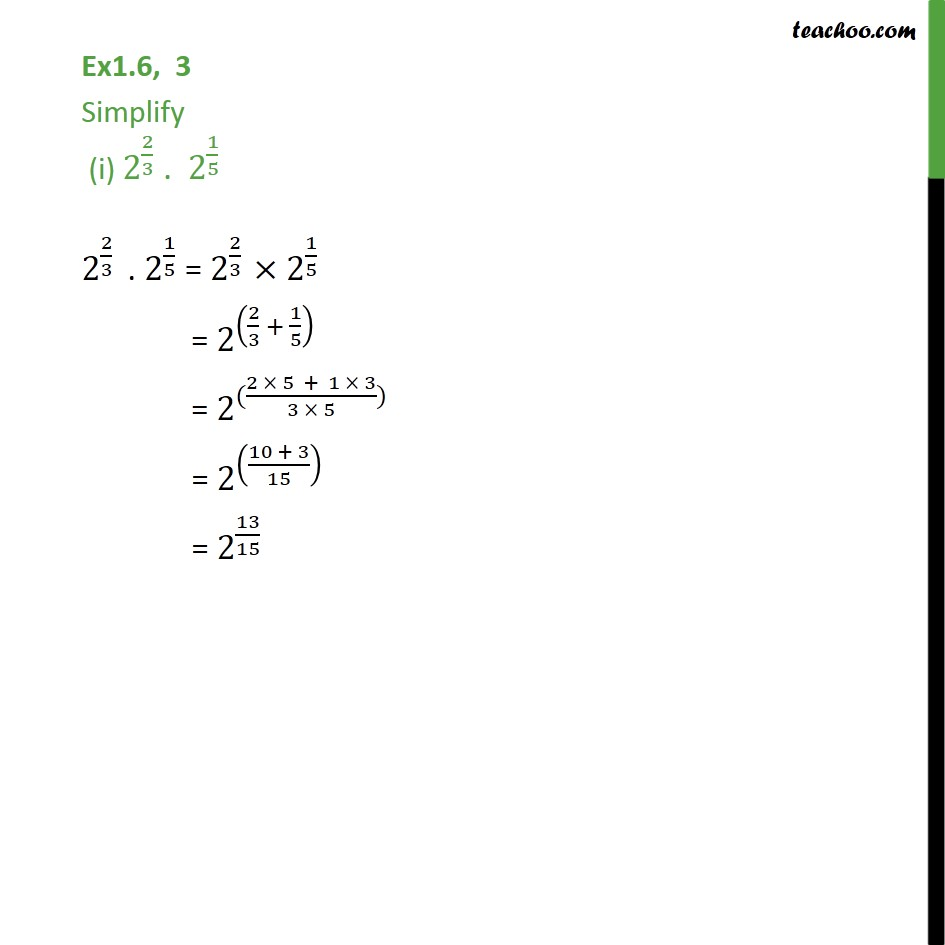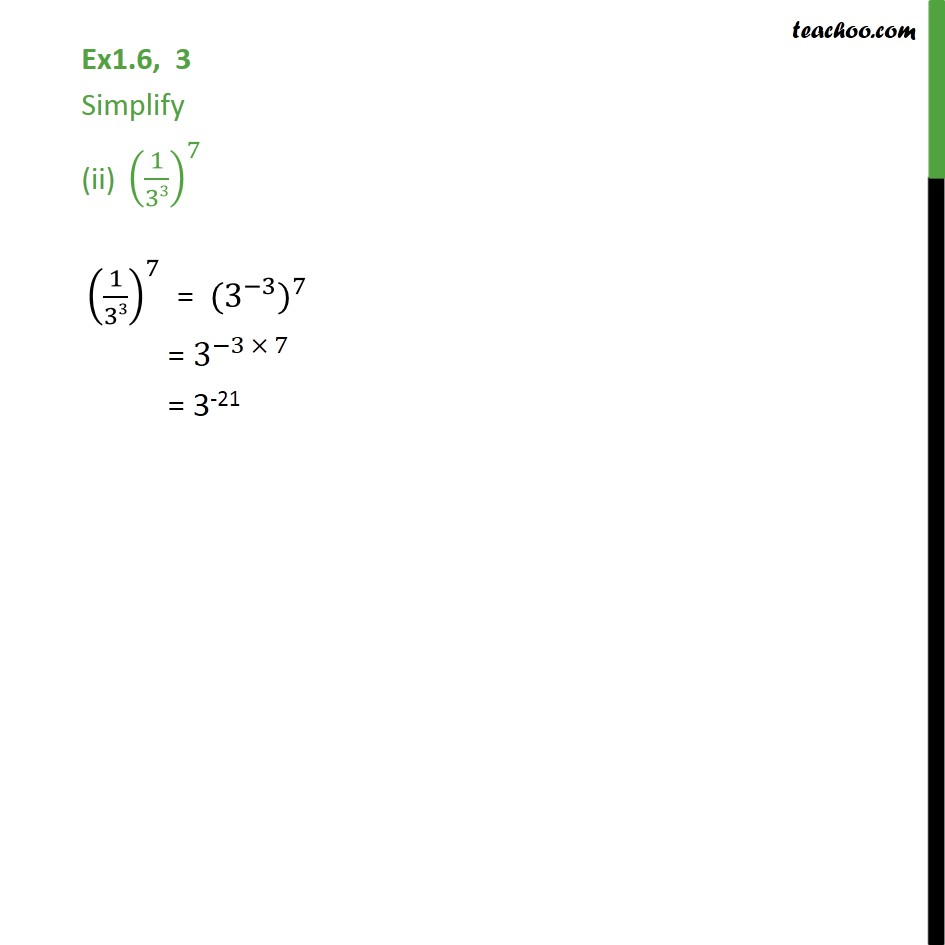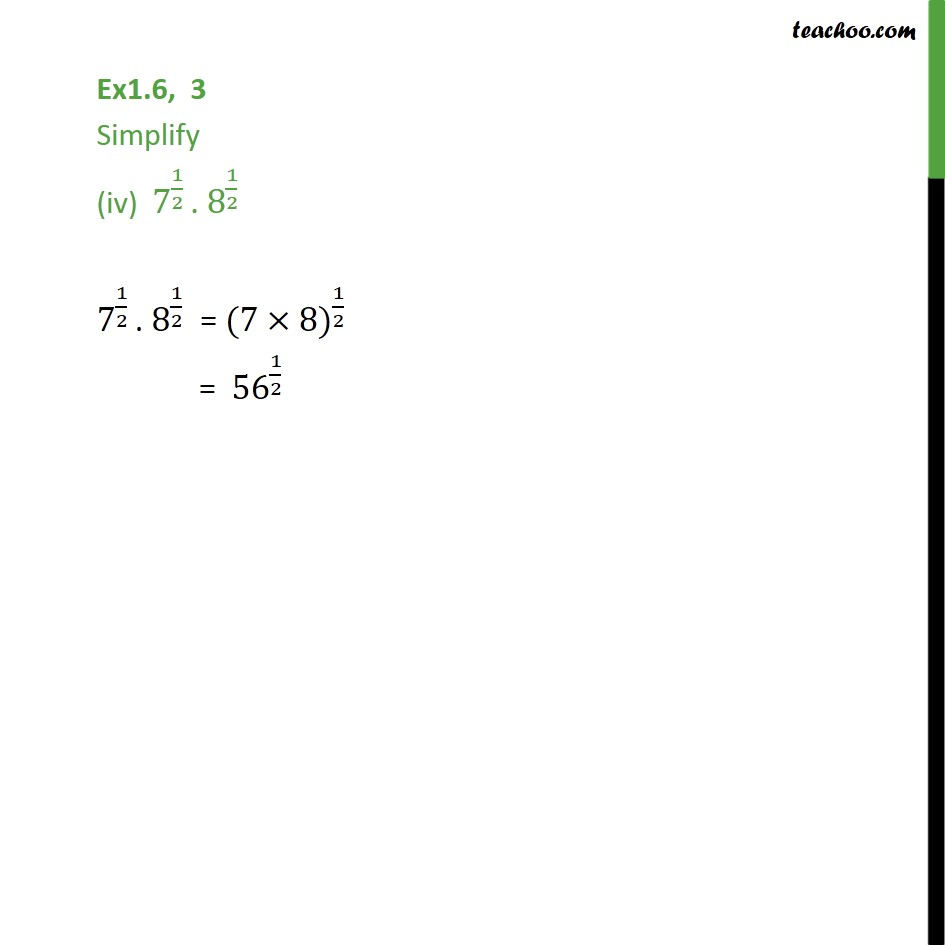Learn All Concepts of Chapter 1 Class 9 - FREE. Check - Chapter 1 Class 9 Maths1. Chapter 1 Class 9 Number Systems
2. Serial order wise
3. Ex 1.6

Transcript

Ex1.6, 3 Simplify (i) 2^(2/3) . 2^(1/5) 2^(2/3) . 2^(1/5) = 2^(2/3) 2^(1/5) = 2^((2/3 + 1/5) ) = 2^(((2 5 + 1 3)/(3 5))) = 2^(((10 + 3)/15) ) = 2^(13/15) Ex1.6, 3 Simplify (ii) (1/33)^7 (1/33)^7 = (3^( 3)) ^7 = 3^( 3 7) = 3-21 Ex1.6, 3 Simplify (iii) 11 ^(1/2)/ 11 ^(1/4) 11 ^(1/2)/ 11 ^(1/4) = 11 ^((1/2 1/4) ) = 11 ^(((1 4 1 2)/(2 4)) ) = 11 ^(((4 2)/8) ) = 11 ^((2/8) ) = 11 ^(1/4 ) Ex1.6, 3 Simplify (iv) 7^(1/2) . 8^(1/2) 7^(1/2) . 8^(1/2) = (7 8) ^(1/2) = 56 ^(1/2)

Ex 1.6